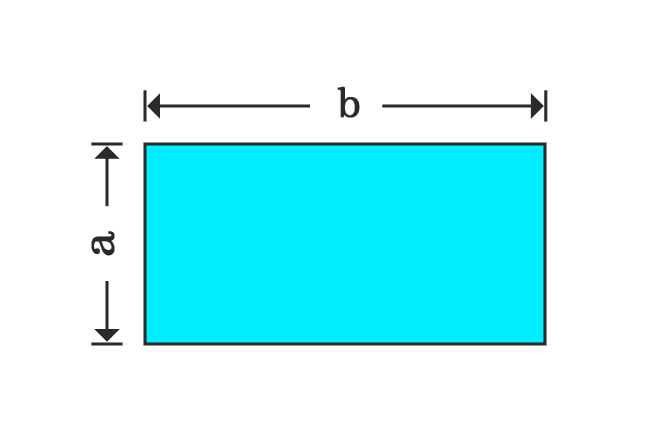# Proof of Distributive property of Multiplication over Subtraction

The distributive property of multiplication over subtraction can be proved in algebraic form by the geometrical method. Actually, it is derived in mathematics by the area of a rectangle.

### Evaluate the Area of RectangleConsider a rectangle but its dimensions are unknown. So, take the width and length of the rectangle as $a$ and $b$ respectively. Now, calculate the area of this rectangle in algebraic form.

$Area \,=\, a \times b$

$\implies$ $Area \,=\, a.b$

$\,\,\, \therefore \,\,\,\,\,\,$ $Area \,=\, ab$

Remember the area of this rectangle and it is used later in proving the distributive property.

### Find the of Areas of Rectangles

Divide the rectangle across its length at a point as two different small rectangles.1. If the length of one rectangle is $c$, then the length of the second rectangle is equal to $b-c$.
2. However, the width of each rectangle is same and it is $a$.

Calculate the area of first rectangle.
$Area \,=\, (b-c) \times a$
$\implies$ $Area \,=\, a \times (b-c)$
$\,\,\, \therefore \,\,\,\,\,\,$ $Area \,=\, a(b-c)$

Calculate the area of second rectangle.
$Area \,=\, c \times a$
$\implies$ $Area \,=\, a \times c$
$\,\,\, \therefore \,\,\,\,\,\,$ $Area \,=\, ac$

### Relationship between the Areas of Rectangles

Geometrically, the first rectangle can be obtained by subtracting the second rectangle from the actual rectangle. So, the area first rectangle is equal to the subtraction of the area of second rectangle from the area of main rectangle.

$\,\,\, \therefore \,\,\,\,\,\,$ $a(b-c) \,=\, ab-ac$

You can derive it in another way if you are confused with the direct geometrical approach.

The area of the rectangle is $ab$ and it is divided as two rectangles whose areas $a(b-c)$ and $ac$. So, the area of the rectangle is equal to the sum of the areas of the small rectangles geometrically.

$ab$ $\,=\,$ $a(b-c)+ac$

According to Transposing method, the term $ac$ can be shifted to left-hand side of the equation from right-hand side.

$\implies$ $ab-ac \,=\, a(b-c)$

$\,\,\, \therefore \,\,\,\,\,\,$ $a(b-c) \,=\, ab-ac$

Latest Math Topics
Jun 26, 2023
Jun 23, 2023

###### Math Questions

The math problems with solutions to learn how to solve a problem.

Learn solutions

Practice now

###### Math Videos

The math videos tutorials with visual graphics to learn every concept.

Watch now

###### Subscribe us

Get the latest math updates from the Math Doubts by subscribing us.